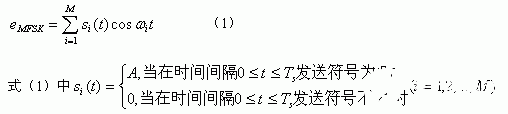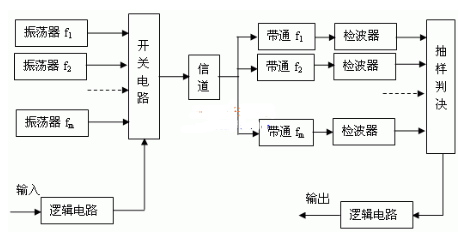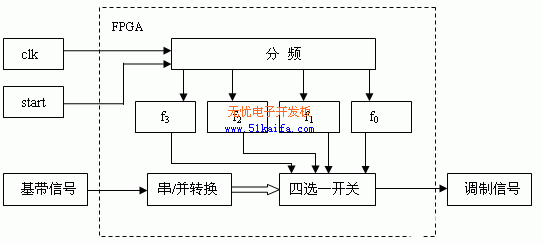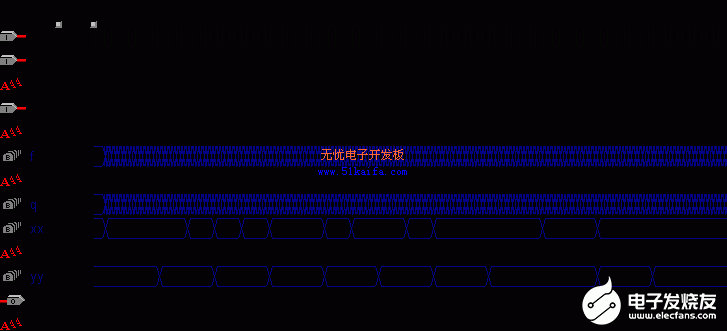# 基于FPGA器件实现多频键控调制电路的设计和仿真验证研究

1、 MFSK简介

MFSK系统是2FSK（二频键控）系统的推广，该系统有M个不同的载波频率可供选择，每一个载波频率对应一个M进制码元信息，即用多个频率不同的正弦波分别代表不同的数字信号，在某一码元时间内只发送其中一个频率。MFSK信号可表示为：2、 MFSK调制电路的FPGA实现

2.1 基于FPGA的MFSK调制电路方框图2.2 MFSK调制电路VHDL程序

entity MFSK is

port（clk ：in std_logic; --系统时钟

start ：in std_logic; --开始调制信号

x ：in std_logic; --基带信号

y ut std_logic）; --调制信号

end MFSK;

architecture behav of MFSK is

signal q ：integer range 0 to 15; --计数器

signal f ：std_logic_vector（3 downto 0）; --分频器

signal xx:std_logic_vector（1 downto 0）; --寄存输入信号x的2位寄存器

signal yy:std_logic_vector（1 downto 0）; --寄存xx信号的寄存器

begin

process（clk） --此进程过对clk进行分频，得到4种载波信号f3、f2、 f1、f0。

begin

if clk‘event and clk=’1‘ then

if start=’0‘ then f elsif f=“1111” then f else f end if;

end if;

end process;

process（clk） --对输入的基带信号x进行串/并转换，得到2位并行信号的yy

begin

if clk’event and clk=‘1’ then

if start=‘0’ then q elsif q=0 then q elsif q=8 then q else q end if;

end if;

end process;

process（clk，yy） --此进程完成对输入基带信号x的MFSK调制

begin

if clk‘event and clk=’1‘ then

if start=’0‘ then y elsif yy=“00” then y elsif yy=“01” then y elsif yy=“10” then y else y end if;

end if;

end process;

end behav;

2.3 仿真结果

MAX+PLUSII环境下的仿真结果如图3所示。3、 结束语

.

0%

0
0%

------分隔线----------------------------